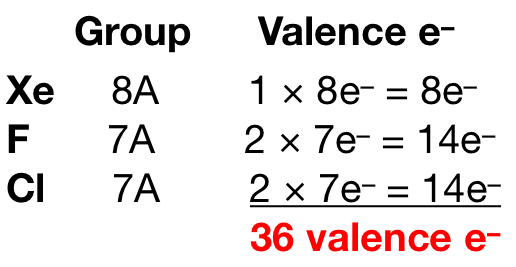Chemistry Practice Problems Dipole Moment Practice Problems Solution: Two different compounds have the formula XeF2Cl2. ...

🤓 Based on our data, we think this question is relevant for Professor Welch's class at UVA.

# Solution: Two different compounds have the formula XeF2Cl2. Write Lewis structures for these two compounds, and describe how measurement of dipole moments might be used to distinguish between them.

###### Problem

Two different compounds have the formula XeF2Cl2. Write Lewis structures for these two compounds, and describe how measurement of dipole moments might be used to distinguish between them.

###### Solution

Xenon (EN = 2.60) is less electronegative than fluorine (EN = 3.98) and chlorine (EN = 3.16) so xenon is the central atom. We then need to count the total number of valence electrons for each element:Fluorine and chlorine prefers to form 1 bond. There are two possible Lewis structures for this compound:View Complete Written Solution

Dipole Moment

Dipole Moment

#### Q. Where, approximately, is the negative pole on each of these molecules?Which molecule should have higher dipole moment, and why?a. COF2 because it cont...

Solved • Tue Jul 09 2019 20:40:56 GMT-0400 (EDT)

Dipole Moment

#### Q. Indicate the bond polarity (show the partial positive and partial negative ends) in the following bond.c. H—Cl

Solved • Mon Nov 26 2018 17:41:30 GMT-0500 (EST)

Dipole Moment

#### Q. Indicate the bond polarity (show the partial positive and partial negative ends) in the following bond.a. C—O

Solved • Mon Nov 26 2018 17:24:41 GMT-0500 (EST)

Dipole Moment

#### Q. State whether or not the following has a permanent dipole moment.

Solved • Mon Nov 26 2018 16:42:09 GMT-0500 (EST)